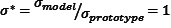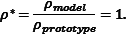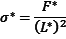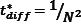# Principles of Centrifuge Modeling

## Typical Applications

A geotechnical centrifuge is used to conduct model tests to study geotechnical problems such as the strength, stiffness and capacity of foundations for bridges and buildings, settlement of embankments, stability of slopes, earth retaining structures, tunnel stability and seawalls. Other applications include explosive cratering, contaminant migration in ground water, frost heave and sea ice. The centrifuge may be useful for scale modeling of any large-scale nonlinear problem for which gravity is a primary driving force.

## Reason for Model Testing on the Centrifuge

Geotechnical materials such as soil and rock have nonlinear mechanical properties that depend on the effective confining stress and stress history. The centrifuge applies an increased “gravitational” acceleration to physical models in order to produce identical self-weight stresses in the model and prototype. The one to one scaling of stress enhances the similarity of geotechnical models and makes it possible to obtain accurate data to help solve complex problems such as earthquake-induced liquefaction, soil-structure interaction and underground transport of pollutants such as dense non-aqueous phase liquids. Centrifuge model testing provides data to improve our understanding of basic mechanisms of deformation and failure and provides benchmarks useful for verification of numerical models.

## Scaling Laws

If a 1 m deep model container is filled with soil, placed on the end of a centrifuge and subject to a centrifugal acceleration of 50 g, the pressures and stresses are increased by a factor of 50. So, the vertical stress at the base of the model container is equivalent to the vertical stress at a depth of 50 m below the ground surface on earth — the 1 m deep model represents 50 m of prototype soil. The reason for the centrifuge is to enable small scale models to feel the same stresses as a full scale prototype. Thisgoal can be stated mathematically as

``

Note the asterisk on a quantity denotes a scale factor for that quantity, i.e. the ratio of the quantity in the model to the quantity in the prototype. σ may represent any quantity with units of pressure (e.g. elastic modulus, shear strength, stress, pressure). Ifthen the stress in the model (smodel) will be equal to the stress in the prototype (sprototype).

With a centrifuge model, we scale down the length (L) of the model and we scale up gravity (g) [or acceleration (a)]. We use the same soil at the same mass density (r) to ensure we get similar behavior. That is, for a given scaling factor N,andNote that stress has units of pressure, or force per unit area. Thus we can writeSubstituting F = m∙a (Newton’s law, force = mass ∙ acceleration) and r = m/L3 (from the definition of mass density),Substituting from above,Therefore, if we build a reduced-scale model using the same soil at the same mass density, reduce the length by a factor of N while simultaneously increasing gravity by the same factor N, then we ensure that the stress in the model is the same as it would be in the prototype.

Scale factors for many other quantities can be derived from the above relationships. The table below summarizes common scale factors for centrifuge testing.

Scale Factors for Centrifuge Model Tests (from Kutter 1992)

a – The diffusion time scale factor depends on whether the diffusion coefficient (e.g., coefficient of consolidation) is scaled. If the same soil is used in model and prototype,Reference: B.L. Kutter, “Dynamic Centrifuge Modeling of Geotechnical Structures”, Transportation Research Record 1336, TRB, National Research Council, pp. 24-30, Washington, D.C., 1992.

## Value of Centrifuge in Geotechnical Earthquake Engineering

Large Earthquakes are infrequent and unrepeatable but they can be devastating. All of these factors make it difficult to obtain the required data to study their effects by post earthquake field investigations. Instrumentation of full scale structures is expensive to maintain over the large periods of time that may elapse between major temblors, and the instrumentation may not be placed in the most scientifically useful locations. Even if engineers are lucky enough to obtain timely recordings of data from real failures, there is no guarantee that the instrumentation is providing repeatable data. In addition, scientifically educational failures from real earthquakes come at the expense of the safety of the public. Understandably, after a real earthquake, most of the interesting data is rapidly cleared away before engineers have an opportunity to adequately study the failure modes.

Centrifuge modeling is a valuable tool for studying the effects of ground shaking on critical structures without risking the safety of the public. The efficacy of alternative designs or seismic retrofitting techniques can compared in a repeatable scientific series of tests.

## Verification of Numerical Models

Centrifuge tests can also be used to obtain experimental data to verify a design procedure or a computer model. The rapid development of computational power over the last two decades has revolutionized engineering analysis. Many computer models have been developed to predict the behavior of geotechnical structures during earthquakes. Before a computer model can be used with confidence, it must be proven to be valid based on experimental data. The meager and unrepeatable data provided by natural earthquakes is usually insufficient for this purpose. Verification of the validity of assumptions made by a computer program is especially important in the area of geotechnical engineering due to the complexity of soil behavior. Soils exhibit highly non-linear behavior, their strength and stiffness depend on their stress history and on the water pressure in the pore fluid, all of which may evolve during the loading caused by an earthquake. The computer models which claim to be able to simulate these phenomena are very complex and require extensive verification. The centrifuge is useful for verifying assumptions made by a computer model. If the results show the computer model to be inaccurate, the centrifuge test data provides some insight into the physical processes which in turn stimulates the development of better computer models.